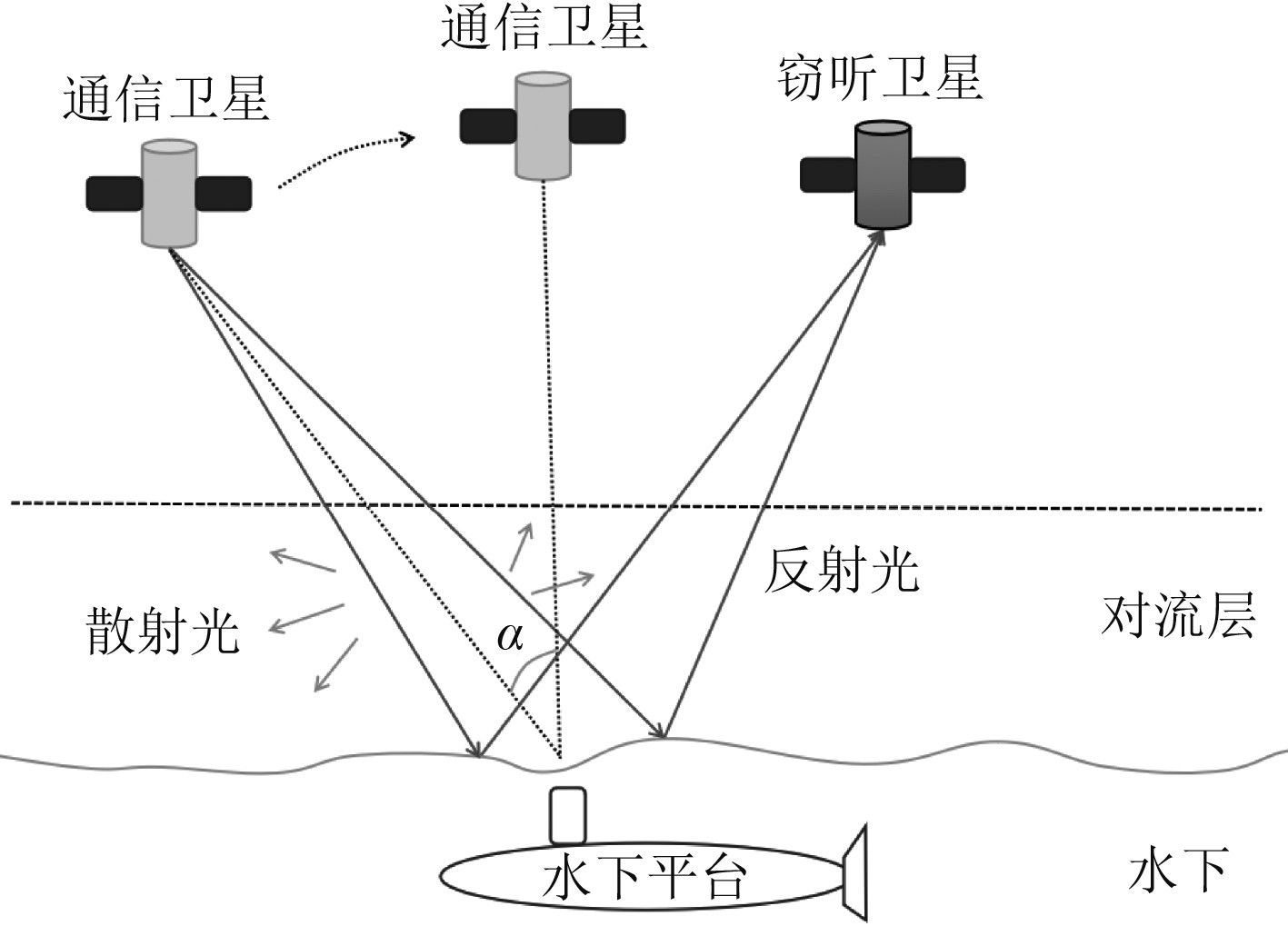﻿ 跨介质对潜激光通信后向散射研究
 舰船科学技术2022, Vol. 44Issue (8): 127-130    DOI: 10.3404/j.issn.1672-7649.2022.08.026PDF

1. 华中科技大学 光学与电子信息学院，湖北 武汉 430205;
2. 中国船舶集团有限公司第七二二研究所，湖北 武汉 430205

Research on backscattering of cross-medium submarine laser communication
LI Ya-ping1,2, WAN Zi-ao2, FEI Li2, CHEN Si-jing2, TANG Ming1, ZHOU Jin-rong2
1. School of Optical and Electronic Information, Huazhong University of Science and Technology, Wuhan 430205, China;
2. The 722 Research Institute of CSSC, Wuhan 430205, China
Abstract: The blue-green laser, as a seawater projection window, has become one of the effective means to solve cross-medium submarine communication by virtue of its lower loss in seawater and its own higher modulation bandwidth. This article focuses on the potential safety hazards of exposure of the position of underwater platforms caused by atmospheric backscattering and sea surface reflection effects in satellite-submarine laser communication. By establishing a layered satellite submarine laser transmission model, the Mie backscattering and reflection effects of the satellite submersible laser under different atmospheric conditions are simulated. Combining the simulation results and the existing optical detection sensitivity, it proves that the underwater platform has the possibility of being located in the satellite-submarine laser communication, providing reference guidance for the subsequent development of equipment and technology.
Key words: laser communication     cross-medium submarine communication     backscattering
0 引　言

1977年，美国国家航空航天局进行了12 km空中平台与海下300 m的水下平台的单向通信试验，证明空中平台与水下平台通信的可行性。1984年，美国海军成功利用卫星激光与1艘名为“海豚”号常规柴油机潜艇进行了对潜通信试验。2016年，贺岩等完成了水下大型作业平台与空中平台间激光通信演示系统。除了实验上的进展，研究人员对跨介质激光通信进行了大量的理论仿真分析，如海洋表面及重力波对激光传输的影响、不同海况下的粗糙海面的透射特性、风速海雾等对激光功率的衰减作用等。然而，由于大气散射效应，部分光子会改变传输方向，进而可能被其他光学侦测设备探测到，造成水下平台位置暴露。

1 大气散射 1.1 大气组成

1.2 大气散射特性

2 大气背向散射仿真模拟

2.1 激光沿任意路径传播衰减

 $T = {{\text{e}}^{{\rm{ - sec}}\alpha \displaystyle\int_{{H_1}}^{{H_2}} {\beta (H){\text{d}}H} }} 。$ (16)

 $N(H) = N(0)\exp \left( - \frac{H}{{{{{H}}_{\text{0}}}}}\right) 。$ (17)表 1 不同能见度对应的标高值 Tab.1 Elevation values corresponding to different visibility

 $\begin{split} & \beta (H) = \beta (0)\exp \left( - \frac{H}{{{H_0}}}\right) ，\\ & {\beta _b}(H) = {\beta _b}(0)\exp \left( - \frac{H}{{{H_0}}}\right)。\end{split}$ (19)

$u = - \sec \theta \displaystyle\int\nolimits_{{H_1}}^{{H_2}} {\beta (H){\rm{d}}H}$ ，将不同层之间衰减累加得到：

 ${{u}} = {\text{ - sec}}\alpha \sum\limits_{{{i}} = 0}^{\rm{n}} {\beta (0){{\rm{e}}^{{\rm{ - }}\frac{{{H_{\rm{i}}}}}{{{H_0}}}}}} \frac{H}{{{n}}} 。$ (20)

 $T = {{\text{e}}^{{\rm{ - sec}}\alpha \sum\limits_{{\text{i}} = 0}^{\text{n}} {\beta (0){{\text{e}}^{{\rm{ - }}\frac{{{H_{{i}}}}}{{M(V)}}}}\frac{H}{{{n}}}} }} ，$ (21)

2.2 仿真过程图 1 跨介质对潜激光通信示意图 Fig. 1 Schematic diagram of cross-medium submarine laser communication

 $n(r) = c{r^2}\exp ( - dr) 。$ (22)

 $c = \frac{{9.781 \times {{10}^{15}}}}{{{V^6}{W^5}}}{\kern 1pt} ，$ (23)
 $b = \frac{{1.304 \times {{10}^4}}}{{VW}} 。$ (24)

 $W = 0.00316{V^{ - 1.54}}，{\rm{g}}/{{\rm{m}}^3}。$ (25)

 $\begin{split} n(r) = &3.104 \times {10^{10}}{V^{1.7}}{r^2}\exp ( - 4.122{V^{0.54}}r) =\\& 5.4 \times {10^7}{W^{ - 1.104}}{r^2}\exp ( - 0.5477{W^{ - 0.351}}r)，{{\rm{m}}^{ - 3}}{\text{μ}}{{\rm{m}}^{ - 1}}。\end{split}$ (26)表 2 不同能见度对应的衰减值和后向散射值 Tab.2 Attenuation value and backscatter value corresponding to different visibility

 ${P_1} = {P_{t1}} + {P_{t2}} 。$ (27)

 ${P_{t1}} = P(1 - T) \cdot \frac{{{\beta _b}}}{{{\beta _{ext}}}} ，$ (28)
 ${P_{t2}} = P{T^2}\varsigma 。$ (29)

 ${P_{probe1}} = {P_{t1}}{(\frac{{{d_1}}}{{{d_2}}})^2}(\frac{\theta }{{360}}) 。$ (30)

 ${P_{probe2}} = {P_{t2}}{(\frac{{H - 12}}{{12}})^3}{\tan ^3}\frac{\theta }{2}{\tan ^3}\alpha 。$ (31)

 ${P_{probe}} = {P_{probe1}} + {P_{probe2}} 。$ (32)图 2 不同能见度下探测光功率与方位角的关系图 Fig. 2 The relationship between the detection optical power and the azimuth angle under different visibility

3 探测回波光子定位水下平台的讨论

4 结　语

  杨坤, 杜度. 国外对潜通信技术发展研究. 舰船科学技术, 2018, 40(1): 153-157. YANG Kun, DU Du. Research on the development of foreign submarine communication technology[J]. Ship Science and Technology, 2018, 40(1): 153-157.  徐涛, 温东, 陈晓露. 基于低轨道卫星的激光星潜双向通信研究[J]. 通信技术, 2016, 49(6): 656-661. XU Tao, WEN Dong, CHEN Xiao-lu. Scheme research on satellite and submarine blue-green laser two-way communication initiated with LEO satellite[J]. Communications Technology, 2016, 49(6): 656-661. DOI:10.3969/j.issn.1002-0802.2016.06.002  DUNTLEY S Q. Light in the Sea[J]. Journal of the Optical Society of America, 1963, 53(2): 214-233. DOI:10.1364/JOSA.53.000214  MCCONATHY D R. Submarine laser communications[C]//Electronics and Aerospace Systems Conference, 1982.  THOMPSON R B, LOPES E F. The effects of focusing and refraction on gaussian ultrasonic beams[J]. Journal of Nondestructive Evaluation, 1984, 4(2): 107-123. DOI:10.1007/BF00566401  贺岩, 周田华, 陈卫标, 等. 水下与空中平台蓝绿激光通信关键技术研究[J]. 科技资讯, 2016, 14(1): 176. DOI:10.3969/j.issn.1672-3791.2016.01.101  KIZILKAYA S. Optical propagation through the ocean surface[D]. The Pennsylvania State University, 2012.  Laser Communication and Propagation through the Atmosphere and Oceans VII. International Society for Optics and Photonics, 2018, 10770: 1077002.  李祥震, 苗希彩, 亓晓. 复杂海况下激光气-海信道传输特性[J]. 光学学报, 2018, 38(3): 243-248.  祖耶夫 B E, 卡巴诺夫 M B. 北京: 光信号在地球大气中的传输[M]. 北京: 科学出版社, 1987.  MCCARTNEY E J. Optics of the atmosphere[J]. Phys Today, 1977.  刘延武, 王红星. 海上激光斜程传输的大气透过率研究[J]. 应用激光, 2010, 30(3): 65-67.  王毅, 饶瑞中. 空间斜程能见度的影响因素分析[J]. 强激光与粒子束, 2003, 15(10): 945-950.  ULABY F T, MOORE R K, FUNG A K. Microwave remote sensing fundamentals and radiometry[C]// Microwave Remote Sensing: Active and Passive. 2010: 5.  RAHMAN A K, ANUAR M S, ALJUNID S A, et al. Study of rain attenuation consequence in free space optic transmission[C]// Telecommunication Technologies 2008 and Malaysia Conference on Photonics. Nctt-Mcp 2008. National Conference on. IEEE, 2009: 64-70.